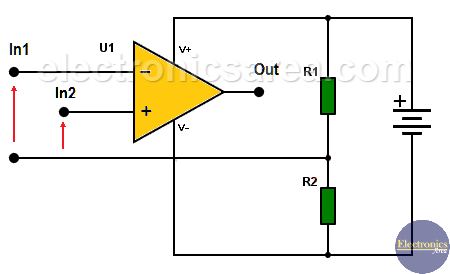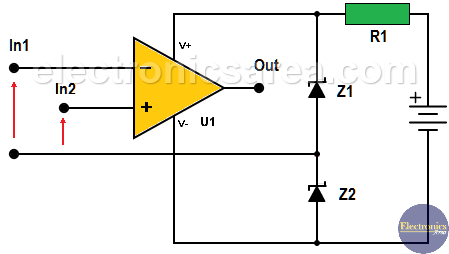# How to use op amps with a single rail power supply?

How to use an Operational Amplifier with a single polarity voltage source? When using an operational amplifier it is necessary to have a double polarity voltage source, one positive and one negative. In this article we are going to learn how to use op amps with a single rail power supply.

To obtain a double polarity voltage source you need to divide the single voltage source into two parts and create an artificial reference point. (the new 0 volts)

There are two widely used methods. One that uses a voltage divider using two resistors and another using zener diodes.

## Method 1: Voltage divider using two resistors

The first figure shows how to obtain the two voltages that an operational amplifier needs from a single polarity voltage source.

A voltage divider has been implemented between the power source terminals, using resistors R1 and R2.

The new reference voltage (0 volts) is obtained from the connection of the resistors. Thus we obtain a positive voltage at the opposite end of R1 and a negative voltage at the opposite end of R2.In DC applications, the resistor values ??R1 and R2 are chosen so that the current passing through them is much greater than the peak output current of the operational amplifier.

This is so, because these resistors are in series with the output of the operational amplifiers.

## Method 2: Voltage divider using two Zener diodes

There are times when the quiescent current passing through R1 and R2 is very high. To avoid this problem they are replaced by zener diodes Z1 and Z2, as shown in the diagram.Zener diodes have a reduced dynamic impedance in series with the output of the operational amplifier, so the quiescent current can be slightly higher than the peak output current and can be adjusted using resistor R1.

As you can see, the value of the resistor R1 and the power of the Zener diodes in the last diagram depend on the amount of current in the output of the operational amplifier.

•
•
•
•
•
•
•
•
•
•
•
•
•
•
•
•
Tagged with: